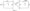# Electronics Engineering Dec-2011

Unit-I

1. (a) State and explain Thevenin’s theorem with example.

(b) For the circuit of figure – 01, write the node voltage equation and determine the current across 10 ohm and 20 ohm resistances of the network.OR

a)  Derive the relationship for converting a 3-terminal star connected resistances into an equivalent delta.

b)  Find the voltage drop between terminal AB, CB and AD in figure = 02.Unit-II

2. a) Prove that in any AC circuit the power consumed by the circuit is P = Vrms 1 rms COS^ – I2 R

b) A 100 ohm resistance is connected in series with choke coil. When a 440 volt, 50 Hz single phase alternating voltage is applied to this combination,* the voltage across the resistance and choke coil are 200 volt and 300 volt respectively. Calculate

i) The resistance and inductance of the coil.

ii) The total resistance and impedance of circuit.

OR

a)  Calculate the active and reactive components of current in each phase of w star connected 1000 Volt, 3-phase generator supplying 50KW at a lagging power factor of 0.8. Find the new output if the current is maintained at the same value with a power factor 0.9 lag.

b) Explain two Wattmeter method of measuring power and power factor. (8)

UNIT – III

3. a) Explain working principle and emf equation of a single phase transformer.

b) A single phase 3300/220 volts, 50 Hz transformer has secondary full load current of 180 Amp. It has 50 turn on its secondary.

Calculate

i) Voltage per turn

ii)  The number of primary turns                                                            –

iii)  The fu 11 load primary current

iv)  The KVA output of the transformer.

OR

a)  Describe the essential parts of DC machines regarding their construction.

b)  An 08 pole DC shunt generator with 778 wave connected armature conductors and running at 500 rpm, supply a load of 12.5 ohm resistance at terminal voltage of 250 volt. The armature resistance is 0.24 ohm and field resistance is 250 ohm. Find the annature current, the induced emf and flux per pole.

UNIT – IV

4. a) Sketch the input and output characteristic curve common – emitter configuration.

b) In a transistor circuit the emitter and collector current are 5.1 m A and 5.0 m A respectively. The collector leakage current with emitter open I is found to be 180 [iA • Calculate the collector leakage current with base open I and /? of the transistor.

OR

a)  Explain static V-I characteristic of SCR.

b)  Unijunction Transistor (UJT) works as a relaxation oscillator, explain with suitable sketches.

UNIT – V

5. a) Derive an equation for the modulated signal and modulation index for amplitude modulation.

b) What is the modulation index of an frequency modulated (FM) carrier having carrier swing of 200 KHz and a modulating signal of 10 KHz.

OR

a)  Discuss the satellite communication system using a diagram.

b) Using simple ray theory describe the mechanism for the transmission of light within an optical fiber.